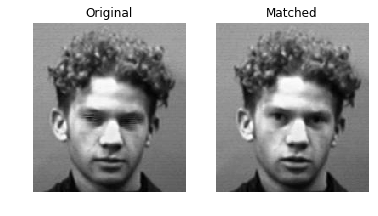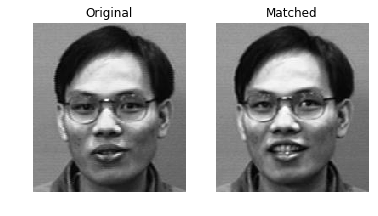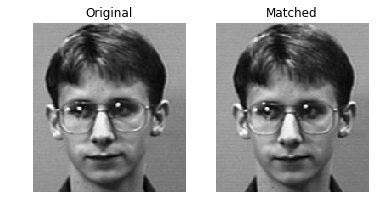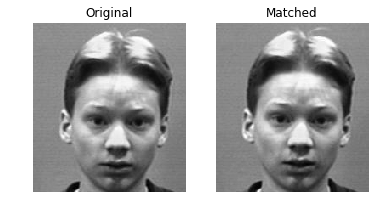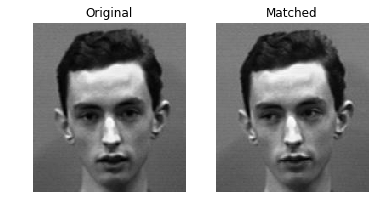# Facial Recognition

Eric Riddoch

Nov 30, 2018

### Contents

This jupyter notebook has the following layout. Items 1-5 build up definitions helper functions, and give a short overview of the mathematical theory behind the algorithm. 6-7 demonstrate the final results.

1. Helper Functions
3. FacialRec Class
4. Mean, Original, and Shifted faces
5. Computing SVD and Eigenfaces
6. Approximating a Face with Eigenfaces
7. Matching a Random Face
In :
import os
import numpy as np
import numpy.linalg as la


# 1. Helper Functions

• get_faces(str):np.array - returns a matrix of all faces in the data set
• sample_faces(int,str):np.array - returns an arbitrary number of randomly selected faces in a matrix
In :
def get_faces(path="./faces94"):
"""Traverse the specified directory to obtain one image per subdirectory.
Flatten and convert each image to grayscale.

Parameters:
path (str): The directory containing the dataset of images.

Returns:
((mn,k) ndarray) An array containing one column vector per
subdirectory. k is the number of people, and each original
image is mxn.
"""
# Traverse the directory and get one image per subdirectory.
faces = []
for (dirpath, dirnames, filenames) in os.walk(path):
for fname in filenames:
if fname[-3:]=="jpg":       # Only get jpg images.
# Load the image, convert it to grayscale,
# and flatten it into a vector.
break
# Put all the face vectors column-wise into a matrix.
return np.transpose(faces)

def sample_faces(k, path="./faces94"):
"""Generate k sample images from the given path.

Parameters:
n (int): The number of sample images to obtain.
path(str): The directory containing the dataset of images.

Yields:
((mn,) ndarray): An flattend mn-array representing a single
image. k images are yielded in total.
"""
files = []
for (dirpath, dirnames, filenames) in os.walk(path):
for fname in filenames:
if fname[-3:]=="jpg":       # Only get jpg images.
files.append(dirpath+"/"+fname)

# Get a subset of the image names and yield the images one at a time.
test_files = np.random.choice(files, k, replace=False)
for fname in test_files:


# 2. Load and Display Functions

The ImageReader class and show() function

In :
from matplotlib import pyplot as plt

"""Class for storing and segmenting images."""

def __init__(self, filename):
"""Read the image file. Store its brightness values as a flat array."""

self.is_gray = len(self.image.shape) == 2
self.gray = None
self.brightness = None

# convert image to grayscale and store as 1D array
if self.image.max() <= 1:
self.image /= 1
else:
self.image = self.image / 255

if self.is_gray:
self.gray = self.image
else:
self.gray = self.image.mean(axis=2)

self.brightness = self.gray.ravel()

def show_original(self):
"""Display the original image."""

# check for color
if len(self.image.shape) == 3:
plt.imshow(self.image)
elif len(self.image.shape) == 2:
plt.imshow(self.image, cmap="gray")

plt.show()

def show_gray(self):
plt.imshow(self.gray, cmap="gray")
plt.show()

def show(image, title="", m=200, n=180):
"""Plot the flattened grayscale 'image' of width 'w' and height 'h'.

Parameters:
image ((mn,) ndarray): A flattened image.
m (int): The original number of rows in the image.
n (int): The original number of columns in the image.
"""

# reshape image
image = image.reshape(m, n)
plt.title(title)
plt.imshow(image, cmap="gray")

# render image
plt.show()

def show_multiple(image_list, title_list, suptitle="", m=200, n=180):
"""Displays multiple pictures in a single row.

Display more than 3 faces at your own peril."""

# create subplots
num_images = len(image_list)
fig, axes = plt.subplots(1, num_images)

fig.suptitle(suptitle)

# plot each image
for i, image in enumerate(image_list):
# reshape images
image = image.reshape(m, n)
axes[i].imshow(image, cmap="gray")
axes[i].set_title(title_list[i])
axes[i].axis("off")

# render images
plt.show()


# 3. FacialRec Class

methods:

• project(np.array,int):np.array - returns the projection of a face onto the subspace spanned by an arbitrary number of input faces
• find_nearest(int,str):np.array - returns an arbitrary number of randomly selected faces in a matrix
• match(int,str):np.array - returns an arbitrary number of randomly selected faces in a matrix
In :
class FacialRec(object):
"""Class for storing a database of face images, with methods for
matching other faces to the database.

Attributes:
F ((mn,k) ndarray): The flatten images of the dataset, where
k is the number of people, and each original image is mxn.
mu ((mn,) ndarray): The mean of all flatten images.
Fbar ((mn,k) ndarray): The images shifted by the mean.
U ((mn,k) ndarray): The U in the compact SVD of Fbar;
the columns are the eigenfaces.
"""
def __init__(self, path='./faces94'):
"""Initialize the F, mu, Fbar, and U attributes.
This is the main part of the computation.
"""
self.F = get_faces(path)
self.mu = np.mean(self.F, axis=1)

# Broadcast subtraction over the COLUMNS.
self.Fbar = self.F - np.vstack(self.mu)
self.U,v,d = la.svd(self.Fbar, full_matrices=False)

def project(self, A, s):
"""Project a face vector onto the subspace spanned by the first s
eigenfaces, and represent that projection in terms of those eigenfaces.

Parameters:
A((mn,) or (mn,l) ndarray): The array to be projected.
s(int): the number of eigenfaces.
Returns:
((s,) ndarray): An array of the projected image of s eigenfaces.
"""
return self.U[:,:s].T @ A

def find_nearest(self, g, s=38):
"""Find the index j such that the jth column of F is the face that is
closest to the face image 'g' with respect to the 2-norm.

Parameters:
g ((mn,) ndarray): A flattened face image.
s (int): the number of eigenfaces to use in the projection.

Returns:
(int): the index of the column of F that is the best match to
the input face image 'g'.
"""
Fhat = self.project(self.Fbar, s)
ghat = self.project(g-self.mu, s)
return np.argmin(np.linalg.norm(Fhat - np.vstack(ghat), axis=0))

def match(self, image, s=38, m=200, n=180):
"""Display an image along with its closest match from the dataset.

Parameters:
image ((mn,) ndarray): A flattened face image.
s (int): The number of eigenfaces to use in the projection.
m (int): The original number of rows in the image.
n (int): The original number of columns in the image.
"""
j = self.find_nearest(image, s)
match = self.F[:,j]

plt.subplot(121)
plt.title("Input Image")
show(image, m, n)

plt.subplot(122)
plt.title("Closest Match")
show(match, m, n)

plt.show()


# 4. Mean, Original, and Shifted faces

### Definitions,

• Mean Face - the face resulting from averaging the grayscale values of every face in the data set
• Shifted Face - subtracting the mean face from a regular grayscale face gives the shifted face

Before performing any facial recognition operations on a face, we "normalize" the face into the shifted face.

### Code Below

1. In FacialRec.__init__(), we compute $F$, the mean face $\boldsymbol{\mu}$, and the mean-shifted faces $\bar{F}$. Then, we store each as an attribute.
2. Initialize a FacialRec object and display its mean face, plus an original image and its shifted face.
In :
# get the j'th face
j = 37

rec = FacialRec()

# get the mean face
mean_face = rec.mu

# get a sample face
face = rec.F[:, j]

# get shifted face
shifted_face = rec.Fbar[:, j]

# display faces
show_multiple([face, shifted_face, mean_face], ["Sample Face", "Shifted Face", "Mean Face"])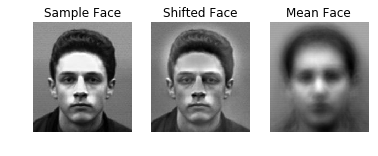# 5. Computing SVD and Eigenfaces

• In FacialRec.__init__(), we compute the compact SVD $(UΣV^{H})$ of $\bar{F}$ and store the $U$ as an attribute. Recall that U, in this case, is an orthonormal basis for the range of $\bar{F}$, and the columns of U are the are ordered from greatest to least importance of any given face.
In :
# show 1'st, 50th, and 100th faces in U
rec = FacialRec()
faces = rec.U[:, [1, 50, 100]]
print("First 3 eigenfaces: there is more of U_0 in every face than any other eigenface")
show_multiple([faces[:, 0], faces[:, 1], faces[:, 2]], ["$U_0$", "$U_1$", "$U_2$"], "Eigenfaces")

First 3 eigenfaces: there is more of U_0 in every face than any other eigenface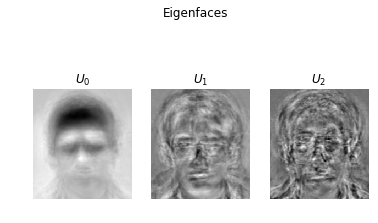# 6. Approximating a Face with Eigenfaces

In the block below, we do the following:

1. Select one of the shifted images $\bar{\mathbf{f}}_i$.
2. Let $s$ be the number of eigenfaces used to reconstruct a face. For 4 values of $s$, we use FacialRec.project() to compute the corresponding $s$-projection $\widehat{\mathbf{f}}_i$. Then we compute the reconstruction $\widetilde{\mathbf{f}}_i$, by adding back the mean face.
3. Display each of the reconstructions and the original image.

Note that by $s = 256$, the face is a perfect approximation.

In :
# get the j'th face
j = 82

rec = FacialRec()

# display j'th face
face = rec.Fbar[:, j]
show(face + rec.mu, "Original Face")

# print 4 reconstructions of varying accuracies
faces = []
names = []
for i in range(1, 5):
names.append(f"s = {i**4}")

# project face
proj = rec.project(face, i**4)

# reconstruct face
recon = rec.U[:, :i**4] @ proj + rec.mu

# show reconstruction
faces.append(recon)

# display approximations
show_multiple(faces[:2], names[:2], r"Eigenface $s$-Approximations")
show_multiple(faces[2:], names[2:])

show_multiple([face + rec.mu, faces, faces], ["", "",  ""],
"Eigenface Reconstruction")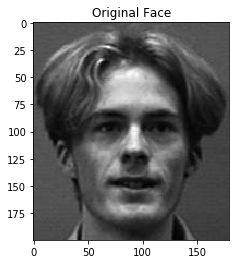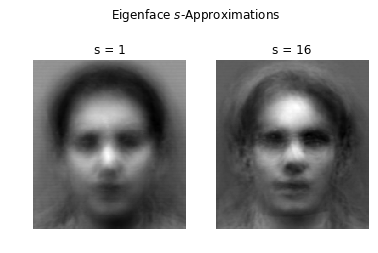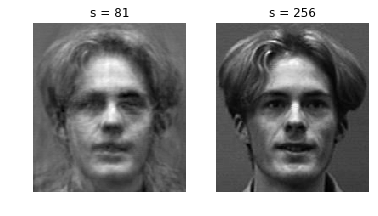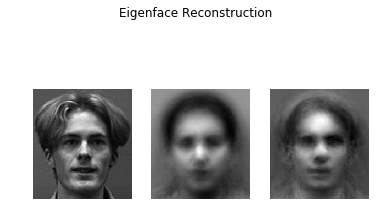# 7. Matching a Random Face

Here we draw a random face, $g$, from the data set and call FacialRec.find_nearest() on it.

By default, this method projects the entire data set of faces onto the space spanned by the first 38 colums of U. Then it returns the face from the set closest to $g$ with respect to the 2-norm.

Observe that the face is almost always identital.

In :
# get a random face from the data set
rec = FacialRec()
rand_index = np.random.randint(0, rec.F.shape - 1)
rand_face = rec.F[:, rand_index]

# match the face in the data set
matched_face_index = rec.find_nearest(rand_face)
matched_face = rec.F[:, matched_face_index]

# display both side by side
show_multiple([rand_face, matched_face], ["Random Face", "Matched Face"])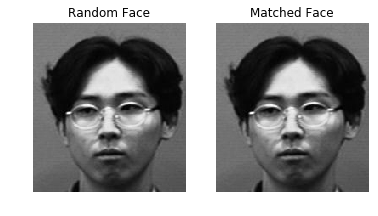And now we do it again 5 times!

In :
n = 5

# draw n faces randomly and loop through them
for i, test_image in enumerate(sample_faces(n)):
# 'test_image' is a now flattened face vector.

# match the face in the data set
matched_face_index = rec.find_nearest(test_image)
matched_face = rec.F[:, matched_face_index]

show_multiple([test_image, matched_face], ["Original", "Matched"])